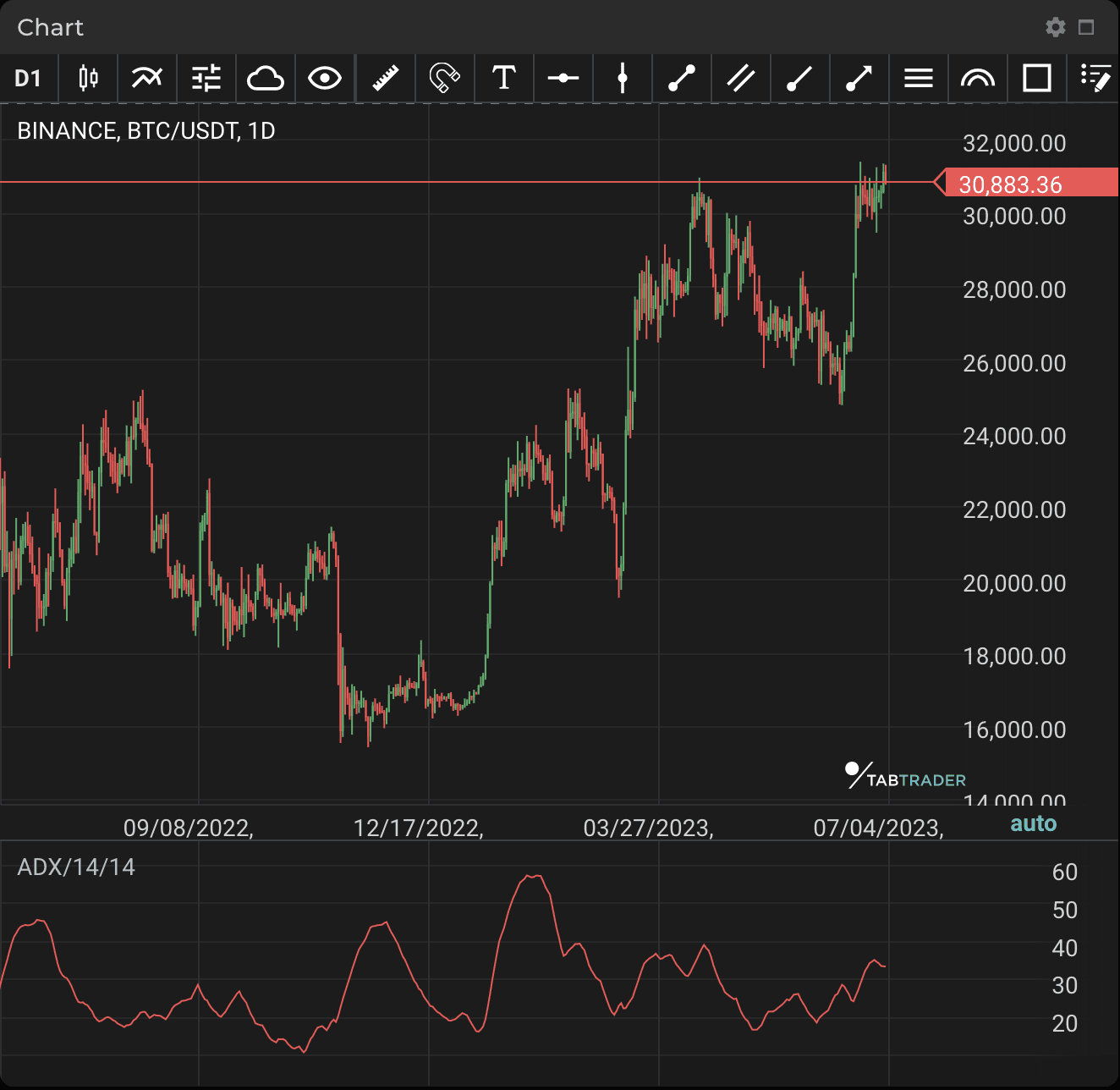# Average Directional Movement Index

Publication date is

The Average Directional Movement Index (ADX) technical indicator helps to determine the strength of a price trend.

ADX is part of a system which attempts to measure the strength of both positive and negative price movement by means of the DMI+ and DMI- indicators along with ADX itself.

ADX calculations are based on a moving average of price range expansion over a given period of time. The default setup is 14 periods (but can be adjusted to your preference in the indicator’s settings).

ADX is presented as a single line within the range of values from a low of 0 to a high of 100. ADX is non-directional; it displays a trend’s strength whether the price is trending up or down.

ADX clearly indicates when the trend is gaining or losing momentum. A series of higher ADX peaks means trend momentum is increasing. A series of lower ADX peaks means trend momentum is decreasing.

Realizing when trend momentum is increasing gives the trader confidence to stay in the position with a view to exiting before the trend reverses. However, a sequence of low-level ADX peaks is a signal to watch price and manage risk.### Calculation

ADX is a combination of the positive directional indicator (+DI) and negative directional indicator (-DI). ADX smoothes the two indicators with a smoothed moving average.

The calculation of +DI and -DI includes the high, low, and closing prices of each period.

One first calculates the directional movement (+DM and -DM):

UpMove = today's high − yesterday's high

DownMove = yesterday's low − today's low

IF UpMove > DownMove and UpMove > 0, then +DM = UpMove, else +DM = 0

IF DownMove > UpMove and DownMove > 0, then -DM = DownMove, else -DM = 0

After selecting the number of periods (14 days was used originally), +DI and -DI are:

+DI = 100x the smoothed moving average of (+DM) divided by average true range -DI = 100x the smoothed moving average of (-DM) divided by average true range

The smoothed moving average is calculated over the number of periods selected, and the average true range is a smoothed average of the true ranges.

Then: ADX = 100x the smoothed moving average of the absolute value of (+DI − -DI) divided by (+DI + -DI).

Looking to get started in crypto trading?

Try TabTrader on mobile or web!